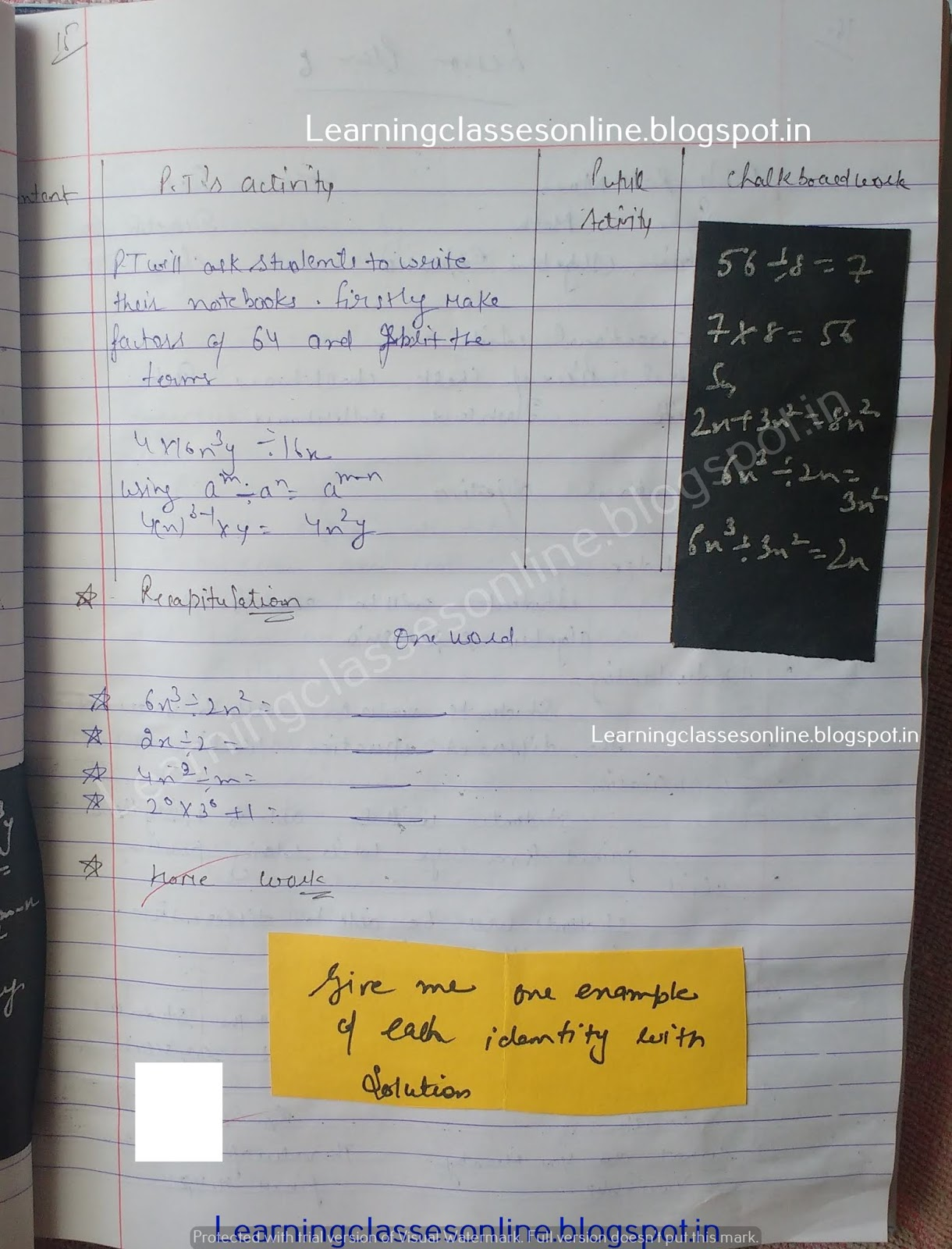Mathematics Lesson Plan on algebra equation and its types

# Mathematics Lesson Plan on algebra equation and its types

Mathematics Lesson Plan on algebra equation and its types for class 6 , 7 and 8 teachers

Note: The Mathematics Lesson Plan given below is just an example. You can change the Name, Class, Course, Date, Duration, etc. according to your needs.Further Reference:
www.learningclassesonline.com Mathematics Lesson Plan for B.Ed